# Engineering Mechanics - Kinematics of Particle (KOP)

### Exercise :: Kinematics of Particle (KOP) - General Questions

• Kinematics of Particle (KOP) - General Questions
6.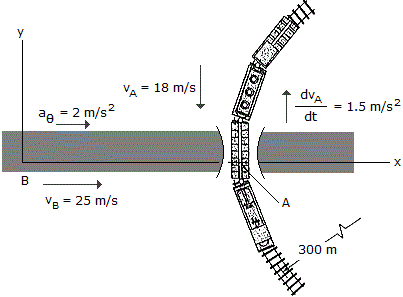A passenger in the automobile B observes the motion of the train car . At the instant shown, the train has a speed of 18 m/s and is reducing its speed at a rate of 1.5 m/s2. The automobile is accelerating at 2 m/s2 and has a speed of 25 m/s. Determine the velocity and acceleration of A with respect to B. The train is moving along a curve of radius = 300 m.

 A. vA/B = (25.0i+18.00j) m/s, aA/B = (2.00i-1.500j) m/s2 B. vA/B = (25.0i+18.00j) m/s, aA/B = (0.920i-1.500j) m/s2 C. vA/B = (-25.0i-18.00j) m/s, aA/B = (-2.00i+1.500j) m/s2 D. vA/B = (-25.0i-18.00j) m/s, aA/B = (-0.920i+1.500j) m/s2

Explanation:

No answer description available for this question. Let us discuss.

7.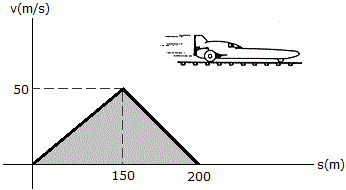The v-s graph for a rocket sled is shown. Determine the acceleration of the sled when s = 100 m and s = 175 m.

 A. a100 = 3.75 m/s2, a175 = -1.250 m/s2 B. a100 = 11.11 m/s2, a175 = -25.0 m/s2 C. a100 = 0.333 m/s2, a175 = -1.000 m/s2 D. a100 = 33.3 m/s2, a175 = -25 m/s2

Explanation:

No answer description available for this question. Let us discuss.

8.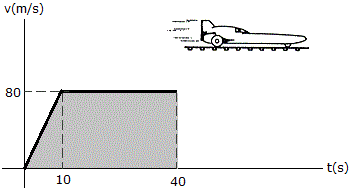From experimental data, the motion of a jet plane while traveling along a runway is defined by the v-t graph shown. Find the position s and the acceleration a when t = 40 s.

 A. s = 2.80 km, a = 2.00 m/s2 B. s = 2.80 km, a = 0 C. s = 2.80 km, a = 2.67 m/s2 D. s = 2.80 km, a = 8.37 m/s2

Explanation:

No answer description available for this question. Let us discuss.

9.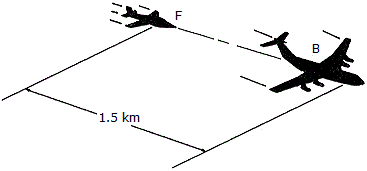The pilot of flighter plane F is following 1.5 km behind the pilot of bomber B. Both planes are originally traveling at 120 m/s. In an effort to pass the bomber, the pilot in F gives his plane a constant acceleration of 12 m/s2. Determine the speed at which the pilot in the bomber sees the pilot of the fighter plane pass at the start of the passing operation the bomber is decelerating at 3 m/s2. Neglect the effect of any turning.

 A. vF/B = 150 m/s B. vF/B = 367 m/s C. vF/B = 90 m/s D. vF/B = 212 m/s

Explanation:

No answer description available for this question. Let us discuss.

10.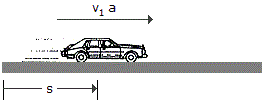A car, initially at rest, moves along a straight road with constant acceleration such that it attains a velocity of 60 ft/s when s = 150 ft. Then after being subjected to another constant acceleration, it attains a final velocity of 100 ft/s when s = 325 ft. Determine the average velocity and average acceleration of the car for the entire 325-ft displacement.

 A. vavg = 80.0 ft/s, aavg = 15.15 ft/s2 B. vavg = 45.2 ft/s, aavg = 13.91 ft/s2 C. vavg = 80.0 ft/s, aavg = 12.57 ft/s2 D. vavg = 55.0 ft/s, aavg = 15.15 ft/s2